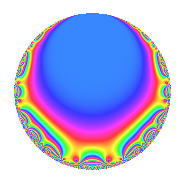# Properties

 Label 354.2.a.gLevel 354 Weight 2 Character orbit 354.a Self dual Yes Analytic conductor 2.827 Analytic rank 0 Dimension 2 CM No Inner twists 1

# Related objects

## Newspace parameters

 Level: $$N$$ = $$354 = 2 \cdot 3 \cdot 59$$ Weight: $$k$$ = $$2$$ Character orbit: $$[\chi]$$ = 354.a (trivial)

## Newform invariants

 Self dual: Yes Analytic conductor: $$2.82670423155$$ Analytic rank: $$0$$ Dimension: $$2$$ Coefficient field: $$\Q(\sqrt{11})$$ Coefficient ring: $$\Z[a_1, \ldots, a_{5}]$$ Coefficient ring index: $$1$$ Fricke sign: $$-1$$ Sato-Tate group: $\mathrm{SU}(2)$

## $q$-expansion

Coefficients of the $$q$$-expansion are expressed in terms of $$\beta = \sqrt{11}$$. We also show the integral $$q$$-expansion of the trace form.

 $$f(q)$$ $$=$$ $$q - q^{2} + q^{3} + q^{4} + ( 1 + \beta ) q^{5} - q^{6} + 4 q^{7} - q^{8} + q^{9} +O(q^{10})$$ $$q - q^{2} + q^{3} + q^{4} + ( 1 + \beta ) q^{5} - q^{6} + 4 q^{7} - q^{8} + q^{9} + ( -1 - \beta ) q^{10} -2 q^{11} + q^{12} + ( -1 - \beta ) q^{13} -4 q^{14} + ( 1 + \beta ) q^{15} + q^{16} -2 \beta q^{17} - q^{18} -2 q^{19} + ( 1 + \beta ) q^{20} + 4 q^{21} + 2 q^{22} + ( 2 - 2 \beta ) q^{23} - q^{24} + ( 7 + 2 \beta ) q^{25} + ( 1 + \beta ) q^{26} + q^{27} + 4 q^{28} + ( -5 - \beta ) q^{29} + ( -1 - \beta ) q^{30} + ( -1 + \beta ) q^{31} - q^{32} -2 q^{33} + 2 \beta q^{34} + ( 4 + 4 \beta ) q^{35} + q^{36} + ( -1 - \beta ) q^{37} + 2 q^{38} + ( -1 - \beta ) q^{39} + ( -1 - \beta ) q^{40} + ( 4 - 2 \beta ) q^{41} -4 q^{42} + 4 q^{43} -2 q^{44} + ( 1 + \beta ) q^{45} + ( -2 + 2 \beta ) q^{46} + ( 2 + 2 \beta ) q^{47} + q^{48} + 9 q^{49} + ( -7 - 2 \beta ) q^{50} -2 \beta q^{51} + ( -1 - \beta ) q^{52} + ( -7 + \beta ) q^{53} - q^{54} + ( -2 - 2 \beta ) q^{55} -4 q^{56} -2 q^{57} + ( 5 + \beta ) q^{58} - q^{59} + ( 1 + \beta ) q^{60} + ( -1 - \beta ) q^{61} + ( 1 - \beta ) q^{62} + 4 q^{63} + q^{64} + ( -12 - 2 \beta ) q^{65} + 2 q^{66} + ( -2 + 2 \beta ) q^{67} -2 \beta q^{68} + ( 2 - 2 \beta ) q^{69} + ( -4 - 4 \beta ) q^{70} + ( 3 + \beta ) q^{71} - q^{72} + ( -2 + 4 \beta ) q^{73} + ( 1 + \beta ) q^{74} + ( 7 + 2 \beta ) q^{75} -2 q^{76} -8 q^{77} + ( 1 + \beta ) q^{78} + ( 1 + \beta ) q^{80} + q^{81} + ( -4 + 2 \beta ) q^{82} -4 q^{83} + 4 q^{84} + ( -22 - 2 \beta ) q^{85} -4 q^{86} + ( -5 - \beta ) q^{87} + 2 q^{88} + ( 2 + 2 \beta ) q^{89} + ( -1 - \beta ) q^{90} + ( -4 - 4 \beta ) q^{91} + ( 2 - 2 \beta ) q^{92} + ( -1 + \beta ) q^{93} + ( -2 - 2 \beta ) q^{94} + ( -2 - 2 \beta ) q^{95} - q^{96} + ( -12 + 2 \beta ) q^{97} -9 q^{98} -2 q^{99} +O(q^{100})$$ $$\operatorname{Tr}(f)(q)$$ $$=$$ $$2q - 2q^{2} + 2q^{3} + 2q^{4} + 2q^{5} - 2q^{6} + 8q^{7} - 2q^{8} + 2q^{9} + O(q^{10})$$ $$2q - 2q^{2} + 2q^{3} + 2q^{4} + 2q^{5} - 2q^{6} + 8q^{7} - 2q^{8} + 2q^{9} - 2q^{10} - 4q^{11} + 2q^{12} - 2q^{13} - 8q^{14} + 2q^{15} + 2q^{16} - 2q^{18} - 4q^{19} + 2q^{20} + 8q^{21} + 4q^{22} + 4q^{23} - 2q^{24} + 14q^{25} + 2q^{26} + 2q^{27} + 8q^{28} - 10q^{29} - 2q^{30} - 2q^{31} - 2q^{32} - 4q^{33} + 8q^{35} + 2q^{36} - 2q^{37} + 4q^{38} - 2q^{39} - 2q^{40} + 8q^{41} - 8q^{42} + 8q^{43} - 4q^{44} + 2q^{45} - 4q^{46} + 4q^{47} + 2q^{48} + 18q^{49} - 14q^{50} - 2q^{52} - 14q^{53} - 2q^{54} - 4q^{55} - 8q^{56} - 4q^{57} + 10q^{58} - 2q^{59} + 2q^{60} - 2q^{61} + 2q^{62} + 8q^{63} + 2q^{64} - 24q^{65} + 4q^{66} - 4q^{67} + 4q^{69} - 8q^{70} + 6q^{71} - 2q^{72} - 4q^{73} + 2q^{74} + 14q^{75} - 4q^{76} - 16q^{77} + 2q^{78} + 2q^{80} + 2q^{81} - 8q^{82} - 8q^{83} + 8q^{84} - 44q^{85} - 8q^{86} - 10q^{87} + 4q^{88} + 4q^{89} - 2q^{90} - 8q^{91} + 4q^{92} - 2q^{93} - 4q^{94} - 4q^{95} - 2q^{96} - 24q^{97} - 18q^{98} - 4q^{99} + O(q^{100})$$

## Embeddings

For each embedding $$\iota_m$$ of the coefficient field, the values $$\iota_m(a_n)$$ are shown below.

For more information on an embedded modular form you can click on its label.

Label $$\iota_m(\nu)$$ $$a_{2}$$ $$a_{3}$$ $$a_{4}$$ $$a_{5}$$ $$a_{6}$$ $$a_{7}$$ $$a_{8}$$ $$a_{9}$$ $$a_{10}$$
1.1
 −3.31662 3.31662
−1.00000 1.00000 1.00000 −2.31662 −1.00000 4.00000 −1.00000 1.00000 2.31662
1.2 −1.00000 1.00000 1.00000 4.31662 −1.00000 4.00000 −1.00000 1.00000 −4.31662
 $$n$$: e.g. 2-40 or 990-1000 Significant digits: Format: Complex embeddings Normalized embeddings Satake parameters Satake angles

## Inner twists

This newform does not admit any (nontrivial) inner twists.

## Atkin-Lehner signs

$$p$$ Sign
$$2$$ $$1$$
$$3$$ $$-1$$
$$59$$ $$1$$

## Hecke kernels

This newform can be constructed as the intersection of the kernels of the following linear operators acting on $$S_{2}^{\mathrm{new}}(\Gamma_0(354))$$:

 $$T_{5}^{2} - 2 T_{5} - 10$$ $$T_{7} - 4$$ $$T_{11} + 2$$